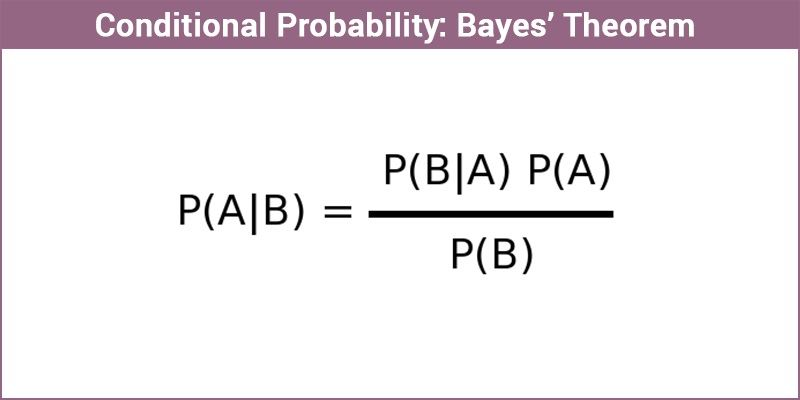# Bayes' Theorem

Bayes’ theorem describes the probability of occurrence of an event related to any condition. It is also considered for the case of conditional probability. For example: if we have to calculate the probability of taking a blue ball from the second bag out of three different bags of balls, where each bag contains three different colour balls viz. red, blue, black. In this case, the probability of occurrence of an event is calculated depending on other conditions is known as conditional probability.## Bayes Theorem Proof

Statement:Let E1, E2,…,En be a set of events associated with a sample space S, where all the events E1, E2,…,En have nonzero probability of occurrence and they form a partition of S. Let A be any event associated with S, then according to Bayes theorem,

$P(E_i│A)~=~\frac{P(E_i)P(A│E_i)}{\sum\limits_{k=1}^{n}P(E_k)P(A| E_k)}$

for any k = 1, 2, 3, …., n

Proof:According to conditional probability formula,

$P(E_i│A)~=~\frac{P(E_i ∩ A)}{P(A)}$ ⋯⋯⋯⋯⋯⋯⋯⋯(1)

Using multiplication rule of probability,
$P(E_i ∩ A)~= ~P(E_i)P(A │E_i)$⋯⋯⋯⋯⋯⋯⋯⋯(2)

Using total probability theorem,
$P(A)~=~\sum\limits_{k=1}^{n}~P(E_k)P(A| E_k)$⋯⋯⋯⋯⋯⋯⋯⋯⋯⋯⋯(3)

Putting the values from equations (2) and (3) in equation 1, we get

$P(E_i│A)~=~\frac{P(E_i)P(A│E_i)}{\sum\limits_{k=1}^n~P(E_k)P(A| E_k)}$

### Bayes Theorem Formula

If A and B are two events, then the formula for Bayes theorem is given by:

 P(A|B) = N(A∩B)/N(B); N(B)≠0

Where P(A|B) is the probability of condition when event A is occurring while event B has already occurred.

N(A ∩ B) is the number of factors common to both A and B.

N(B) is the number of factors in B

### Examples and Solutions

Some illustrations will improve the understanding of the concept.

Example 1:Bag I contains 4 white and 6 black balls while another Bag II contains 4 white and 3 black balls. One ball is drawn at random from one of the bags and it is found to be black. Find the probability that it was drawn from Bag I.

Solution:Let $E_1$ be the event of choosing the bag I, $E_2$ the event of choosing the bag II and A be the event of drawing a black ball.

Then,$P(E_1)~ = ~P(E_2)~ =~\frac{1}{2}$

Also,$P(A|E_1) ~= ~P$(drawing a black ball from Bag I) = $\frac{6}{10}~ = ~\frac{3}{5}$

$P(A|E_2) ~=~ P$(drawing a black ball from Bag II) = $\frac{3}{7}$

By using Bayes’ theorem, the probability of drawing a black ball from bag I out of two bags,

$P(E_1 |A)~ =~\frac{P(E_1)P(A|E_1)}{P(E_1 )P(A│E_1 )+ P(E_2)P(A|E_2)}$

=$\large\frac{\frac{1}{2}~\times~\frac{3}{5}}{\frac{1}{2}~\times~\frac{3}{7}~+~\frac{1}{2}~ ×~\frac{3}{5}}$ = $\frac{7}{12}$

Example 2:A man is known to speak truth 2 out of 3 times. He throws a die and reports that number obtained is a four. Find the probability that the number obtained is actually a four.

Solution:Let $A$ be the event that the man reports that number four is obtained.

Let $E_1$ be the event that four is obtained and $E_2$ be its complementary event.

Then, $P(E_1)$ = Probability that four occurs = $\frac{1}{6}$

$P(E_2)$ = Probability that four does not occurs = $1 ~–~ P(E_1) ~=~ 1~-\frac{1}{6}~ =~\frac{5}{6}$

Also, $P(A|E_1)$ = Probability that man reports four and it is actually a four = $\frac{2}{3}$

$P(A|E_2)$ = Probability that man reports four and it is not a four = $\frac{1}{3}$

By using Bayes’ theorem, probability that number obtained is actually a four,

$P(E_1 |A)~$ $= \large \frac{P(E_1)P(A|E_1)}{P(E_1 )P(A│E_1 )~+~ P(E_2)P(A|E_2)}~ =~\frac{\frac{1}{6} ~ ×~ \frac{2}{3}}{\frac{1}{6} ~×~ \frac{2}{3}~ +~ \frac{5}{6}~ ×~\frac{1}{3}}$
= $\frac{2}{7}$

Students, are you struggling to find a solution to a specific question from Bayes theorem? We will make it easy for you. For detailed discussion on the concept of Bayes’ theorem, download BYJU’S – the learning app

#### 1 Comment

1. Chenna

Outstanding explanation